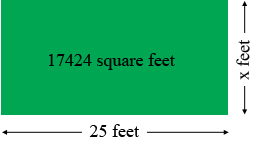SEARCH HOMEMath Central Quandaries & QueriesQuestion from Micheal a student: If one side of .4 acre is 25 feet what would the other three sides beHi Micheal,

The first thing I did was to type 0.4 acres in square feet into the Google search window. Google responded with 0.4 acre = 17424 square feet.

If the properly is a rectangle, 25 feet along the front and $x$ feet along the side then the back is also 25 feet long and the remaining side is $x$ feet long.Thus $25 \times x = 17425.$ Solve for $x.$

If the property is not a rectangle Then you need more information.Math Central is supported by the University of Regina and The Pacific Institute for the Mathematical Sciences.